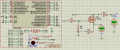#### datdang

Joined Feb 21, 2023
23I have an electrical circuit in the above image. When I turn on Digital Pin 3 (IO3) of Arduino to switch on Mosfet IRF3205 ,the voltage values measured at Drain and Source pin of Mosfet IRF3205 are 1.5V and 0.79V respectively as simulated using Proteus . But in practice , when I connected Arduino and Mosfet on test board like the electrical circuit in Proteus file , the voltage value at Mosfet Drain when Digital Pin 3 is turned on is not 1.5V, it was reduced to 0.2V which leads to voltage at Mosfet Source around 0 V.

My aim is to measure exactly the voltage at Source pin of Mosfet to calculate the resistor R8, it works well with Proteus simulation but in practice it’s so different . I heard once that Proteus doesn’t simulate correctly the operation of Arduino ???

The following text is the code uploaded to Arduino .

void setup()

{ // put your setup code here, to run once:

pinMode(3, OUTPUT);

}

void loop()

{ // put your main code here, to run repeatedly:

digitalWrite(3, HIGH);

delay(1000);

digitalWrite(3, LOW);

delay(1000);

}

#### StefanZe

Joined Nov 6, 2019
178
Hi,
I don't think your circuit will work. What is the PIN AD0 doing? If it is an analog input, there is (almost) no current flowing through your resistor and mosfet. No current through a resistor means no voltage (U=R*I).

#### crutschow

Joined Mar 14, 2008
32,842
That is a standard MOSFET which does not work properly with the low gate voltages you have.
The snip from its data sheet below shows that the Vgs(th) , where it just starts to turn on, can be as high as 4V, and it needs Vgs=10V to fully turn on.
You need a logic type MOSFET with a max Vgs(th) of ≤2V.

Also reduce the value of R7 to no more than 100Ω and increase R6 to 100kΩ.•twohats

#### datdang

Joined Feb 21, 2023
23
That is a standard MOSFET which does not work properly with the low gate voltages you have.
The snip from its data sheet below shows that the Vgs(th) , where it just starts to turn on, can be as high as 4V, and it needs Vgs=10V to fully turn on.
You need a logic type MOSFET with a max Vgs(th) of ≤2V.

Also reduce the value of R7 to no more than 100Ω and increase R6 to 100kΩ.

View attachment 294868
I changed R7=47ohm, R6=100kohm, R5=47ohm,R8=470ohm, and I connected gate of Mosfet to 12V DC, the voltage measured at Vs is 0.84 V, which is not correct . it Should be Vs=Vd(1.56V)*470/517=1.41V ???

#### Alec_t

Joined Sep 17, 2013
13,769
The output pin of the Arduino can source only a few mA, so could be damaged if the FET source resistor has a low value.

#### BobTPH

Joined Jun 5, 2013
7,507
What is that circuit supposed to do?

MOSFETs are rarely used as anything but switches, in which case you want Vds to be as low as possible. For an N-channel MOSFET, the source is usually connected directly to ground.